Hands-On Deep Learning Example - Combine | Combine

### Hands-On Deep Learning Example

So you want to become a Data Scientist? You have followed the online tutorials step-by-step and gotten your first neural networks trained and performing well on the dataset you were given. Now you want to try to do something on your own.. but how? Here we will go through an example process of setting up your first very own deep learning problem and how to solve it. All the way from data collection to final model.

#### The Problem

In this blog we are going to create a model which counts the number of fingers (1-5) a hand is holding up based on a picture of that hand. First we need to collect data, this data will then need to be processed to a suitable format for deep learning. Once the data is ready, we will train a deep convolutional neural network and make a basic interface which shows the results in real-time.

#### Collecting Data

For our problem we need pictures of a hand holding up different numbers of fingers and corresponding labels. The labels should be the number of fingers the hand is holding up. For this purpose we will need a camera, more specifically a webcamera which we can connect to our computer. We don’t want to overcomplicate the problem right away, therefore we want to keep the data very consistent so let’s take all the pictures with a plain white background, for example a white desk, or perhaps a white wall. We want to place our camera at a distance so the hand fills the majority of the image, the ideal distance will depend on your camera but between 20-30 cm away from your plain white surface should be about right.

Our camera is now in place and we are ready to start taking pictures.. but we soon realize two problems. First, we don’t want to have to take potentially hundreds of pictures manually one-by-one, and secondly, we will need to somehow label the data with the correct label (i.e. number of fingers shown). Of course we could take a picture, and manually give it the correct label, but this process would be very time consuming. Let us automate the process instead. In this blog we will use the CV2 python library. This library will let us capture images from our camera from a python script, solving the first problem of having to manually take pictures.

We make a script which uses the CV2 library to capture an image from our camera, we will also take care of the labelling problem by telling the user how many fingers to hold up. Using the CV2 library we show the current image on screen, as well as some text telling the user how many fingers they should be holding up. Using a loop we can then take multiple pictures and for each one we will automatically label it with how many fingers we told the user to hold up (this assumes that the user follows the instructions correctly). Once we have taken a good amount of pictures, we let the user know its time to hold up a different amount of fingers. The pseudo-code of the process is shown below.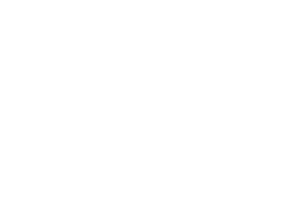Figure 1: Pseudo code for collecting data

For fast reading and writing to disk, and to simplify later on, we recommend saving the data as a numpy array and not as images.

#### Data Pre-processing

We now have our images with corresponding label. We will now process the data so it can be used for training of a neural network. The first thing we need to do is to one-hot-encode our labels, if you have encountered classification problems before this should be familiar. Next we want to convert our images to gray-scale. In this problem we are not interested in any feature related to colour so we can work with gray-scale images instead to reduce the complexity. Next, we want to rescale our image pixel values, for example to lie between 0-1. Lastly, we will resize the image to reduce the complexity further (64×64 pixels).

We can either make a separate script which does the pre-processing, our we can add it to our data collection script, reducing the disk space and the need of running two different scripts. The pseudo-code for data collection + data pre-processing is shown below.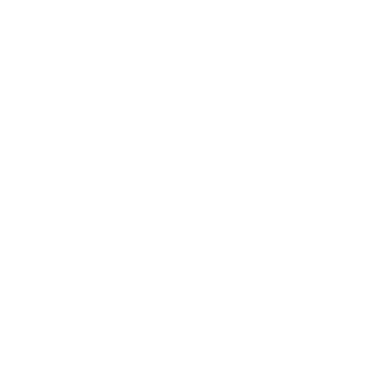Figure 2: Pseudo code for collecting and pre-processing data

Here we also save all the images and labels together in one large numpy array instead of each of separately.

#### Training a model

With our data ready we can now define and train a model. Since we are working with images we will need a convolutional neural network. Our output should be a class, i.e. how many fingers are being held up. One excellent choice of framework for defining and training a neural network is Keras. With this toolbox we can easily create our network exactly as we want it and Keras will take care of all the difficult mathematical operations in the background. Below is the pseudo-code for defining our network, and training it on our data.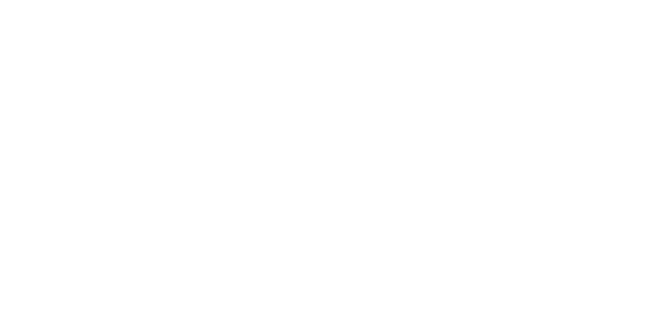Figure 3: Pseudo code for collecting and pre-processing data

The exact architecture of the network, how much dropout is applied and activation functions can be altered to get the best performance on your dataset.

#### Showing the results

With our trained model, the last step is to make a simple interface to try out or model. For this we can revisit the CV2 library we used for data collection. Simply take a picture, run it through our trained model and show the picture + the resulting class from our model on screen. The pseudo-code and an example of how it can look is shown below.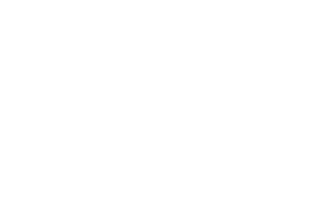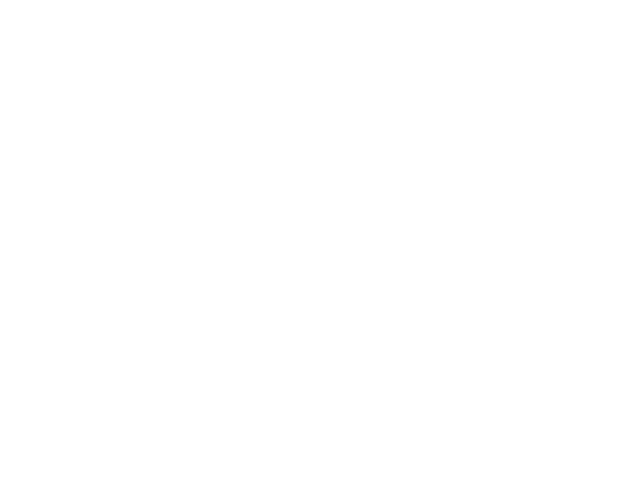Figure 4: Showing the results